A quadratic equation is a second-order Polynomial(1)

with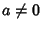. The rootscan be found by Completing the Square:(2)(3)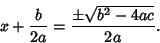(4)

Solving forthen gives(5)

An alternate form is given by dividing (1) through by: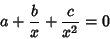(6)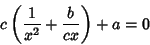(7)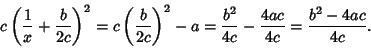(8)

Therefore,(9)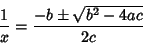(10)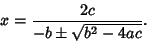(11)

This form is helpful if, in which case the usual form of the Quadratic Formula can give inaccurate numerical results for one of the Roots. This can be avoided by defining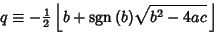(12)

so thatand the term under the Square Root sign always have the same sign. Now, if, then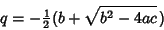(13)(14)

so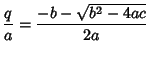(15)(16)

Similarly, if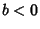, then(17)(18)

so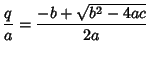(19)(20)

Therefore, the Roots are always given byand.

See also Carlyle Circle, Conic Section, Cubic Equation, Quartic Equation, Quintic Equation, Sextic Equation

References

Abramowitz, M. and Stegun, C. A. (Eds.). Handbook of Mathematical Functions with Formulas, Graphs, and Mathematical Tables, 9th printing. New York: Dover, p. 17, 1972.

Beyer, W. H. CRC Standard Mathematical Tables, 28th ed. Boca Raton, FL: CRC Press, p. 9, 1987.

Courant, R. and Robbins, H. What is Mathematics?: An Elementary Approach to Ideas and Methods, 2nd ed. Oxford, England: Oxford University Press, pp. 91-92, 1996.

King, R. B. Beyond the Quartic Equation. Boston, MA: Birkhäuser, 1996.

Press, W. H.; Flannery, B. P.; Teukolsky, S. A.; and Vetterling, W. T. Quadratic and Cubic Equations.'' §5.6 in Numerical Recipes in FORTRAN: The Art of Scientific Computing, 2nd ed. Cambridge, England: Cambridge University Press, pp. 178-180, 1992.

Spanier, J. and Oldham, K. B. The Quadratic Function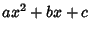and Its Reciprocal.'' Ch. 16 in An Atlas of Functions. Washington, DC: Hemisphere, pp. 123-131, 1987.